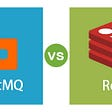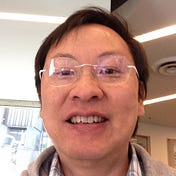# QC — Quantum Algorithm with an example

In Part 5 & 6, we look into the details of both single and 2-qubit quantum gates — the building blocks for quantum algorithms. Let’s get ready to build a quantum program. In this example, we will demonstrate ways to build a quantum program, in particular how quantum parallelism and interference work. The interference plays a key role in creating a pattern of measurements to calculate the final results.

# Deutsch-Jozsa Algorithm

Deutsch-Jozsa algorithm is one of the first quantum algorithms with nice speedup over its classical counterpart. Consider this as my “hello world” in quantum computing but don’t expect it as a single line program.

Consider a function f that takes 0 or 1 as input and outputs either 0 or 1.

Our functions in mind are either balanced or constant. A function f is called balanced if it outputs 0 half the time and 1 the other half. It is a constant function if its output is a constant (1 or 0) regardless of input. Here is an example with a single-bit input.

Our task is given a function, determine it is constant or balanced. The problem is simple but involves a few cool quantum concepts. But before we develop the maths, let’s conceptualize a possible solution.

For classical computing, we can query the function twice and aggregate the result with some if-then-else statements.

If the number of input bits is increased to n, to verify a function is balanced, we query f for

Nice. We have a problem with query complexity that can grow exponentially in the worst case. Let’s expand n to 2 qubits. We can use Hadamard gates to prepare a superposition representing all 4 possible bit combinations. We assume that we have an Oracle function (a super smart or a super slick quantum circuit). It uses the quantum parallelism concept to compute all values of f(x) from the superposition in polynomial time, instead of growing exponentially with the number of bases. Let’s not bother on how this oracle function is implemented for now. It deserves some separate discussion at the end of the article.

By creating a superposition, we allow an Oracle function to work on all possible configurations all at once.

The key idea is the Oracle function will output values that after applying interference (a transformation), we will measure 0 for all constant functions or 1 for balanced functions.

# Single bit input

Let’s lay down a big picture before detailing it. For simplicity, our function f will take 0 or 1 only (a single-bit input). Below is the quantum circuit. Step ① and ② prepares the superposition, step ③ is the oracle and step ④ is the interference.

To realize what is happening exactly, let’s do some math. The corresponding math corresponding to each step above is:

① applies a Pauli X-gate to the second qubit which converts it from |0⟩ to |1⟩.

② applies a Hadamard gate to each of the qubits and put them into a superposition

Now, our first qubit is in a superposition representing both possible input |0⟩ and|1⟩ for the Oracle function. ③ applies an oracle function

For f(x) = 0, the second qubit is simply y. (For simplicity, we will drop 1/√2 from the equation for now.)

For f(x) = 1, we flip the sign.

By examining both outputs, we can generalize the equation as:

Replacing |x⟩ with |0⟩ +|1⟩, we get

Here is the result after applying the Oracle function.

We can go through all possible combination of f and compute the superposition of the first qubit for each scenario.

We find that for the same function type, the values differ by a sign only. i.e. by a global phase with the Δγ equals π.

Superpositions different by a constant global phase are indistinguishable in the real word. They always have the same probability of measurements regardless of how they measure. This does not change as long as no operations or the same operations are applied to both.

This is excellent news! Within the same function type, they will always measure the same way. So our focus is applying a transformation so different groups end with different measurements. ④ applies the Hadamard gate and a constant function will be measured as |0⟩ and a balanced function as |1⟩.

# Multiple-bit input

Let’s expand our solution for input with n bits (say f(1101)). We can apply n Hadamard gates to prepare the superposition.

And apply the Oracle function again. If you work out the case where f(x)=0 and f(x)=1, like what we did, you will end up the same equation in the last step below.

The second qubit above will remain the same for the rest of the circuit. So for simplicity, we just focus on the first qubit.

Then, we apply n Hadamard gate again. The general equation for the Hadamard gate transformation on multiple qubits is:

This is just a nice condensed formularization (nothing new) where <x , y> is the bitwise dot product of the binary representation of x and y. For example,

So after applying Hadamard gates, the qubits become

Let see the case when y = |00000…00⟩. Now, x · y = 0.

It becomes:

When f(x) is balanced, half of the inner summation terms above will destructively cancel each other. If f(x) is a constant, all the inner summation terms will add up together and becomes

So for the former case, no measurement should be made for |00000…00⟩ while in the second case, all the measurements are |00000…00⟩. In short, the measurement of the superposition depends on the interference created by f(x).

Yes. We are done with the first “hello world”. Let’s recap the math before we see the quantum circuit.

# Recap

Here is a quick recap of the whole calculation:

# Implementation

Let’s implement it. The Oracle function here represent the function:

Since we know the exact function we want to support, the quantum circuit can be simplified and implemented as:

Here are the measurements running on a real quantum computer.

Measurements for |000⟩ are very low. Mostly caused by gate errors and noise. So the Oracle function is a balanced function. That is exactly the type of our function belonging to.

Let’s have an oracle representing a constant function. Actually, an identical operator will do it.

As expected, we measure the three qubits as |000⟩.

# Oracle function

Deutsch-Jozsa Algorithm and many other quantum algorithms provide a solution template for a large range of problems. By replacing the Oracle function with a domain-specific function, we can apply the same kind of solution to many problems. Unfortunately, this creates a major stumbling block when first studying quantum computing. The speedup expectation is raised too high with the illusion that we break the curse of the exponential problem forever — the solution may be hard but generally available. In our example, we present an Oracle that hardcode the knowledge of the function into the circuit. We do not query the value of f(x) directly. We map the inputs directly to the output we want. I call it slick because the solution is not generalized enough for any practical use. People want to know the magic of not query f exponentially and we only provide some toy examples. So the disappointment is well understood.

The reality is if we expect the Oracle function to handle any possible functions, query the function for at least half of the possible data seems unavoidable for the worst case. We can argue that the information is already available in superposition so we can compute them in parallel. It is not that simple and we will not elaborate it further.

The real-life problems that can beat the classical algorithms must discover a pattern that can load information into superposition efficiently or they only need a polynomial amount of information. In our toy example, we discover a pattern of the function and take advantage of it. But such hardcoded knowledge hurts its generalization. So what is the point if we do not show a real problem with a nice Oracle implementation? We will continually discover generic algorithms based on Oracle functions to learn different quantum tricks. In the process, we will learn all the methods and combine them to solve significant problems. Shor’s algorithm is one good example which is used to break RSA cryptography.

Quantum computing is not the answer to everything. Scientists are focusing on the BQP problems below. We know prime factoring and many problems belong to this category. So we are looking into what other problems may belong to this group of the algorithm.

# Next

We will look into a few more Oracle based algorithms to get familiar with quantum algorithms before we get into Shor’s algorithm.

--

--

--

## More from Jonathan Hui

Deep Learning

Love podcasts or audiobooks? Learn on the go with our new app.

## Microservices Myths## Kubernetes, Microservices, and Github Actions Deployments## Python→Redis→RabbitMQ## With Visual Studio 2022 you can eliminate Resharper## Echo Pass Season 2 Launch## Summary of Device Coverage Report 2021Deep Learning

## Understanding The Derivative Of The Sigmoid Function## Naïve Bayes vs. SVM for Image Classification## Image-Template matching using Cross-Correlation## Sequence to Sequence Learning With Neural Networks To Perform Number Addition